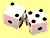## Weakness of Monte Carlo Simulation

• Monte Carlo simulation was originally developed to study properties of equilibrium system. It is not universally acceptable whether this method can also be used to simulate system that is not in equilibrium (i.e. transient state)
• To use Monte Carlo Simulation, you need to generate large number of sampling. This can be time consuming to reach the desired results
• You cannot generate only single sample and use it as the result of simulation. You need to generate many samples and get the average (or any other statistics) as the results.
• The results of Monte Carlo Simulation are only an approximation of the true value, not exact
• Large Variance may be produced by the simulation

Preferable reference for this tutorial is

Teknomo, Kardi. Monte Carlo Simulation Tutorial. http://people.revoledu.com/kardi/ tutorial/Simulation